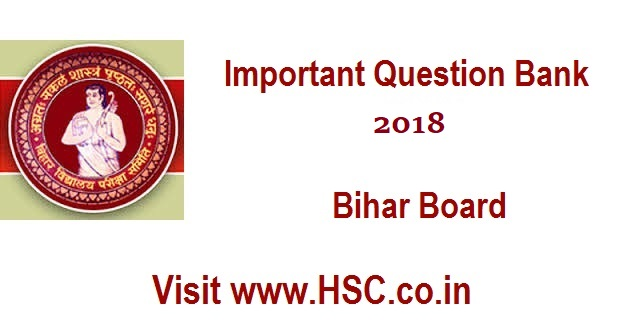# Physics  – 51 Important Question Bank for Bihar Class 12th Intermediate (HSC) Board Exam 2018

HSC Board Exams are fast approaching and students are getting anxious about how to prepare for their HSC Board Exams. So we had mentioned some HSC Study Tips to help students in Cracking HSC Exams.

After the tremendous success of our last year Important Questions Bank for Bihar Class 12 Intermediate (HSC) Board Exam 2016 and Important Questions Bank for  Bihar Class 12 Intermediate (HSC) Board Exam 2017 we have also created a list of Most Important Questions Bank for  Bihar Class 12 Intermediate (HSC) Board Exam 2018 which are likely to appear in HSC Board Exams this year.To unlock the content Click on any of 3 buttons available in the box below saying “This content is Locked”. Once you click on the button the content will get unlocked on same page itself. You must click on social media button showing in below box ie Facebook, Twitter or Google Plus to unlock the content.

1. What are the characteristics of LASER rays?
2. State and explain different types of Telescope. With the help of a neat and labelled diagram explain the working and magnifying power of an Astronomical telescope.
3. State Ampere’s circuital law. Express it mathematically.
4. What do you mean by Wheatstone bridge? Discuss its balanced condition.
5. Draw a neat and labelled diagram of human eye. Discuss the various types of defect of vision briefly?
6. State Brewster’s law. Express it mathematically.
7. Define amplitude modulation and discuss the production of amplitude modulation.
8. State the factors by which the range of transmission of TV signal can be increased.
9. Derive the expressions for the de-Broglie wavelength.
10. What is nuclear fission reaction? Mention its nuclear reaction which take place in nuclear reactor?
11. For a prism, explain deviation and establish the relation Delta=A(n-1)
12. Two lenses, one diverging of power 2 diopters and the other converging of power 6 diopters are combined together. Calculate the focal length and power of the combination.
13. Explain the working principle of a transformer. Explain with the help of a labelled diagram.
14. Calculate the radius of a nucleus of mass no.8.
15. Explain the application of p-n- junction diode as rectifier.
16. Explain Biot-Savart law. State its applications.
17. Explain with the help of neat and labelled diagram the principle of Davison and Germer experiment.
18. Define and deduce the general expression of mutual inductance.
19. Explain Faraday’s law of electromagnetic induction and discuss Lenz correction.
20. What do you mean by Photoelectric effect? State and derive Einstein’s Photoelectric equation?
21. State Kirchhoff’s two laws of electrical network.
22. Derive an expression for energy stored in a capacitor.
23. What are the different components of generator? Explain with the help of diagram.
24. State and explain refraction through convex spherical surfaces.
25. State the properties of Photons.
26. As astronomical telescope having a magnifying power of 8 consists of two thin lenses 45cm apart. Find the focal length of the lenses.
27. State and explain Lens maker’s formula.
28. Define electric field intensity and write its SI unit with dimension also.
29. Give two applications of ultra-violet waves.
30. State and Prove Gauss’s theorem.
31. Write the N-type and P-type semiconductors.
32. Differentiate between interference and diffraction?
33. What is the lens of Presbyopia and Astingmatism?
34. Explain the transistor as a switch and transistor as an amplifier.
35. What are the advantages of LED?

36. What are the limitations of cyclotron?
37. With the help of mean and labelled diagram explain the construction, working principle and sensitivity of moving coil galvanometer? Mention its conversion into ammeter and voltmeter.
38. Name the principle on which optical fibre works. Mention its applications.
39. Define parallel plate capacitor. Explain its construction and working principle of parallel plate capacitor. Derive an expression for its capacitance?
40. What are the constructive and destructive interference. Also find its expression.
41. Deduce an expression for parallel plate capacitor with dielectric slab?
42. Verify laws of reflection or laws of refraction on the basis of Huygen’s wave theory.
43. Find the electric field on the axial line of electric field.
44. Define Potentiometer. With the help of potentiometer compare the e.m.gs of two cells and measure internal resistance of the cell.
45. Give postulates of Bohr’s theory. Give hydrogen spectrum on the basis of Bohr’s theory.
46. The image of a candle is formed on a screen by a convex lens. It half of lens is blacked then what will be the size of image?
47. A wire is stretched 50%, calculate % change in its resistance.
48. Express the general formula of Joule’s heating effect of current.
49. State the different types of losses in a transformer.
50. Express the relationship between commercial unit of energy and SI unit of energy.
51. A carrier wave of peak voltage 12V is used to transmit a message signal. What should be the peak voltage of the modulating signal in order to have a modulation index of 75%?

Hi, we’re trying to collate and gather the data and would be updating it here a few days before the exam. Please keep on visiting our website for updates.

Please use the comments box below and post questions that you think are important from your analysis. It would help the HSC community a lot.

Do subscribe to our updates so that you do not miss out on any important information that we push your way.

Don’t forget to read : MUST REMEMBER THINGS on the day of Exam for HSC Students